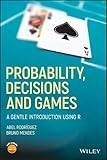# Probability, Decisions and Games: A Gentle Introduction using R9781119302605

Probability, Decisions and Games: A Gentle Introduction using R

By 作者: Abel Rodríguez - Bruno Mendes
ISBN-10 书号: 1119302609
ISBN-13 书号: 9781119302605
Edition 版本: 1
Release Finelybook 出版日期: 2018-04-24
Pages 页数: 240

INTRODUCES THE FUNDAMENTALS OF PROBABILITY, STATISTICS, DECISION THEORY, AND GAME THEORY, AND FEATURES INTERESTING EXAMPLES OF GAMES OF CHANCE AND STRATEGY TO MOTIVATE AND ILLUSTRATE ABSTRACT MATHEMATICAL CONCEPTS
Covering both random and strategic games, Probability, Decisions and Games features a variety of gaming and gambling examples to build a better understanding of basic concepts of probability, statistics, decision theory, and game theory. The authors present fundamental concepts such as random variables, rational choice theory, mathematical expectation and variance, fair games, combinatorial calculus, conditional probability, Bayes Theorem, Bernoulli trials, zero-sum games and Nash equilibria, as well as their application in games such as Roulette, Craps, Lotto, Blackjack, Poker, Rock-Paper-Scissors, the Game of Chicken and Tic-Tac-Toe. Computer simulations, implemented using the popular R computing environment, are used to provide intuition on key concepts and verify complex calculations.
The book starts by introducing simple concepts that are carefully motivated by the same historical examples that drove their original development of the field of probability, and then applies those concepts to popular contemporary games. The first two chapters of Probability, Decisions and Games: A Gentle Introduction using R feature an introductory discussion of probability and rational choice theory in finite and discrete spaces that builds upon the simple games discussed in the famous correspondence between Blaise Pascal and Pierre de Fermat. Subsequent chapters utilize popular casino games such as Roulette and Blackjack to expand on these concepts illustrate modern applications of these methodologies. Finally, the book concludes with discussions on game theory using a number of strategic games. This book:
Features introductory coverage of probability, statistics, decision theory and game theory, and has been class-tested at University of California, Santa Cruz for the past six years
Illustrates basic concepts in probability through interesting and fun examples using a number of popular casino games: roulette, lotto, craps, blackjack, and poker
Introduces key ideas in game theory using classic games such as Rock-Paper-Scissors, Chess, and Tic-Tac-Toe.
Features computer simulations using R throughout in order to illustrate complex concepts and help readers verify complex calculations
Contains exercises and approaches games and gambling at a level that is accessible for readers with minimal experience
Adopts a unique approach by motivating complex concepts using first simple games and then moving on to more complex, well-known games that illustrate how these concepts work together
About the Companion Website
Chapter 1:An Introduction to Probability
Chapter 2:Expectations and Fair Values
Chapter 3:Roulette
Chapter 4:Lotto and Combinatorial Numbers
Chapter 5:The Monty Hall Paradox and Conditional Probabilities
Chapter 6:Craps
Chapter 7:Roulette Revisited
Chapter 8:Blackjack
Chapter 9:Poker
Chapter 10:Strategic Zero-Sum Games with Perfect Information
Chapter 11:Rock-Paper-Scissors:Mixed Strategies in Zero-Sum Games
Chapter 12:The Prisoner's Dilemma and Other Strategic Non-zero-sum Games
Chapter 13:Tic-Tac-Toe and Other Sequential Games of Perfect Information
Appendix A:A Brief Introduction toR
Index
End User License Agreement

Probability, Decisions and Games A Gentle Introduction using R 9781119302605.epub

Probability, Decisions and Games A Gentle Introduction using R 9781119302605.pdf

### 觉得文章有用就打赏一下文章作者

#### 支付宝扫一扫打赏#### 微信扫一扫打赏• QQ咨询
• 回顶